• 검색 결과가 없습니다.

# Inferring the physical 3D shapes of early galaxies

N/A
N/A
Protected

Share "Inferring the physical 3D shapes of early galaxies"

Copied!
52
0
0
더 보기 ( 페이지)

전체 글

An observational challenge is that we only observe the 2D projections of galaxies in the sky, while we are interested in actually inferring the 3D shapes of galaxies. We find that we can accurately recover 3D shapes for large samples of galaxies (N>100) that follow a Gaussian distribution in their 3D properties, given realistic observational uncertainties. In the second part of the thesis we contributed to the development of a new photometry tool, called ForcePho, to measure fluxes and the 2D shapes for a large number of galaxies observed with the James Webb Space Telescope (JWST).

The main assumption of this code is that the galaxies can be described with Sérsic profiles. Panel on the left is the result with φ = 0 and panel on the right is the result with φ=π/2. The number of data fed into the MCMC model is the number of galaxies * the number of models (= 2000) * the number of Markov chains (= 4).

We can see here that as the uncertainty of the input increases, the uncertainty of the output also increases. 12 9 The shape of the fake galaxy with half a beam of light = 1.0 arcsecond, the galaxy follows the.

The Cosmological Framework

Formation and Evolution of Galaxies

## New era with the James Webb Space Telescope

By detecting the ultraviolet radiation emitted by the first stars and galaxies, the secrets of the population of the first stars and galaxies are revealed.

Recent works with Deprojection

## Constraining 3D Shapes from 2D Projection

JWST has its own purpose of studying physics, such as the Lyman continuum escape fraction, that lies behind the cosmic reionization. The coordinate (x', y', z') is the coordinate where z' is the line of sight, x' lies inside the x-y plane. Therefore, the rotation matrix becomes R = AB. sinφ −cosφcosθ cosφsinθ cosφ −sinφcosθ sinφsinθ.

From the relationship we can now obtain the equation of the projected ellipse by adding f, g, h to the equation. Finally, Euler's theorem  is used to find the major and minor axes of this projected ellipse. The matrix equation of the projected ellipse is a2= 1. 12) By solving the eigenvector-eigenvalue equation from the characteristic equation of the projected ellipse, we can obtain the major and minor axes.

Therefore, the ratio of the axes of the projected ellipse is q≡b′. 14) Finally, we can now know how observed data recorded in 2D can be converted into true 3D forms.

The outline of the thesis

Ideas of the Projection Function

## Bayesian Hierarchical Modeling

Bayes' theorem is to create new conditions using the prior and the probability functions of the prior. The model we are considering is that the Bayesian probability with events is stochastically independent. This likelihood function, the prior function, and the recommended distribution are the inputs to Numpyro.

The parameters for the catalog are the five variables of the projection function a,ξ (b/a),ζ(c/a), theta, phi and also the result of the projection function a' and b'. The only important point is that xi is always greater than zeta from Eq. The number of data is defined as the multiplication of the number of iterations (number of mock galaxies), the number of samples and the number of chains.

The number of galaxies may differ in the calculation, but we have limited the number of samples and the number of Markov chains in this calculation to 2000 and 4. The idea of ​​full pooling is that we can think of each variable as having the same values, or that the values ​​have their representatives. We can predict that the full pooling produced results with more center-centered and small uncertainties.

The triaxial values ​​a, ξ and ζ are fitted simultaneously for all galaxies (i.e. assumed to be the same for one galaxy in the sample), while the two projection angles are assumed to be galaxy-specific (i.e. vary from galaxy to galaxy ). The idea behind this model is to make each variable separate for each galaxy. By changing the input data, a difference in the number of spurious galaxies or a difference in the input uncertainties can lead to a different result.

The errors (16–84 percentiles of the score) are compared with the number of galaxies, the level of input uncertainty and the number of input data kept in the MCMC calculation. This procedure can check the Bayesian hierarchical model or the confidence level of the model.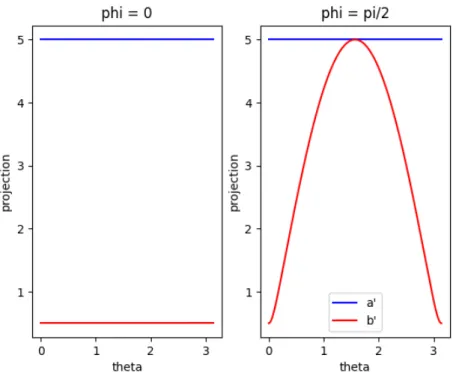Figure 3: The projection function for the disk shaped galaxy. a’ and b’ is a semi major and semi minor axis for projected ellipses from the ellipsoid

## ForcePho, the Photometry tool for JWST

It first models the galaxy from the target Sérsic index and half the light radius. We measure the residual of both, analyze the Gaussians that were added to the model.

## Result of the Bayesian Hierarchical model

The comparison of the two full coupling and no coupling models in Figure 7 shows how much they are different. Full pooling may show almost exactly the same as the true value, however the unpooled result is showing a scatter from the true value. When the number of model galaxies, or the iteration of the calculation increases, the difference between the true value and the mean of the calculation result decreases.

Of course, the overall pooling score has low output uncertainty, the 16th and 84th percentiles are almost the same as the median score. In addition, the difference between the true value and the median of the calculation result increases when. The difference between the true value and the median of the total repentance score results in more drastic changes, because complete clustering calculates the variables as their groups.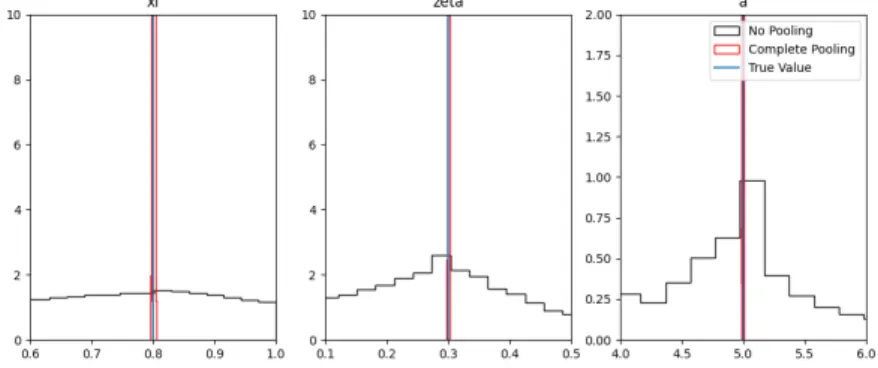Figure 6: The comparison result for the complete pooling model and the no pooling model

## ForcePho

This is a limitation of the current code, which does not cover all areas of the galaxy model.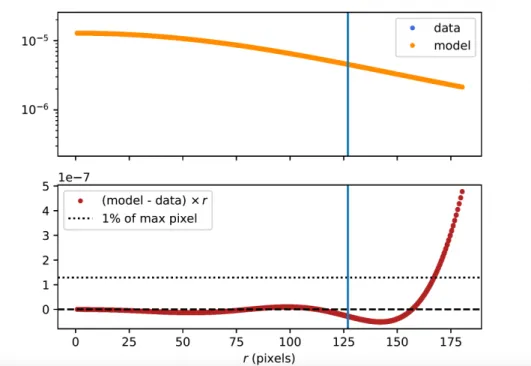Figure 10: The second part’s result of the ForcePho code. Top figure is the plotting of the input and the model function

Summary

Outlook for the Future Works

Code for the ForcePho

## Code for the Bayesian Hierarchical Model

Binney, "Testing for triaxiality with kinematic data," Monthly Notices of the Royal Astronomical Society, vol. Robertson, "Galaxy Formation and Reionization: Key Unknowns and Expected Breakthroughs by the Ijames Web Space Telescope/i," Annual Review of Astronomy and Astrophysics, vol. Dekel, “Radiative feedback and the low efficiency of galaxy formation in low-mass haloes at high redshift,” Monthly Notices of the Royal Astronomical Society , vol.

First of all, I want to thank Professor Sandro Tacchella, who guided me into the world of cosmic dawn and the first galaxies. And Professor Moses, although I do not have many relations with you, thank you for joining my graduate committee. Thanks for being my friend and helping me with tons of problems made from Ulsan.

수치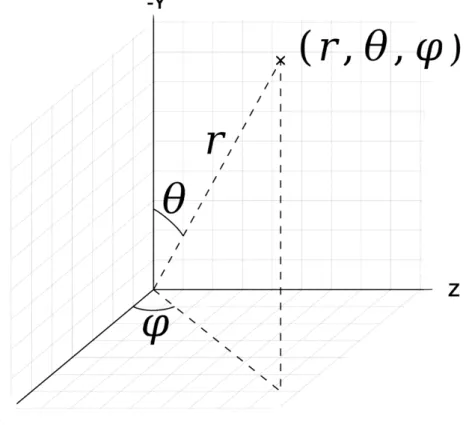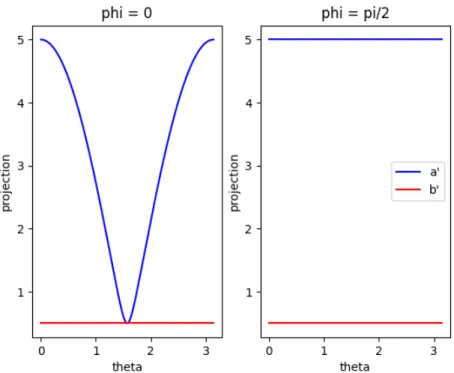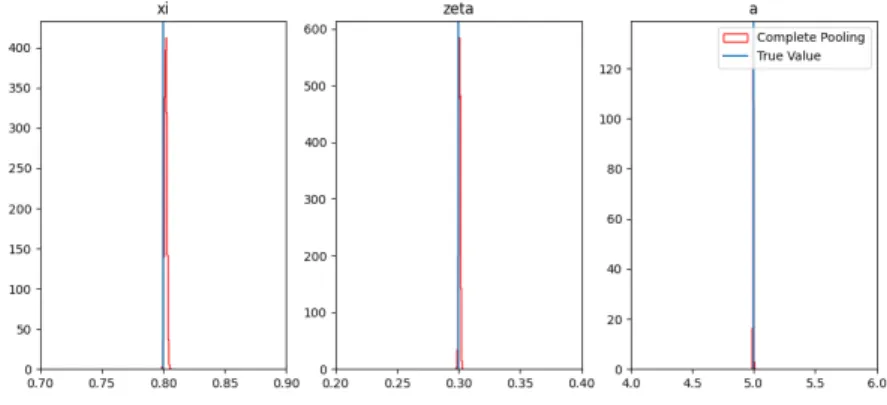+7

참조

관련 문서

This calculation follows a general method for finding torus charts on Hori–Vafa mirrors to complete intersections in toric varieties, detailed in  generalising the method of

• 대부분의 치료법은 환자의 이명 청력 및 소리의 편안함에 대한 보 고를 토대로

Pooling Cross-section and Time Series Data in the Estimation of a Dynamic Model: The Demand for Natural Gas. Econometric Analysis of Panel Data, second

Genetic parameter estimates of meat quality traits in Duroc pigs selected for average daily gain, longissimus muscle area, backfat thickness, and intramuscular

In section 2, we provide the establish the exponential in- equalities for sum of extended acceptable random variables and in section 3, we obtain a result dealing with

• Nitric acid was used as leaching agent; the influence of acidity, solid to liquid ratio, leaching time, and agitation rate on REEs extrac- tion was investigated.. The recovery

75. Papachristou GI, Muddana V, Yadav D, et al. Comparison of BISAP, Ranson’s, APACHE-II, and CTSI scores in predicting organ failure, complications, and mortality

The 9 infants were referred for dysphagia evaluation at 1 of the 3 study sites, where they underwent a complete instrumental evaluation. All infants met the following 3 cri- teria:

1 IfAbe a complete discrete valuation ring of characteristic 0 with perfect residue field of characteristicp, then it is a p-ring... LetAbe a strict p-ring with residue ringk, and

언어 선택하기

웹사이트는 선택한 언어로 번역됩니다.

기타 언어